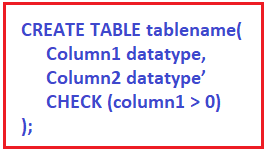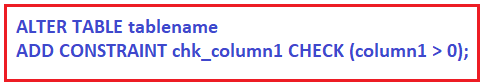# Check Constraints in MySQL

## Check Constraints in MySQL with Examples

In this article, I am going to discuss CHECK Constraints in MySQL with Examples. Please read our previous article where we discussed UNIQUE Constraint in MySQL. At the end of this article, you will understand everything about the MySQL CHECK Constraints.

##### Check Constraints in MySQL

The MySQL Check Constraint is used to enforce domain integrity. Domain integrity means the values that are going to store in a table column must be followed by some defined rules such as range, type, and format. In other words, we can say that Check Constraint ensures the valid entries for a given column value by restricting the type of the value, the format of the data, or the range of possible values.

The CHECK Constraint in MySQL can be applied to single or multiple columns of a table. The CHECK Constraint in MySQL can be defined while creating a new table or using ALTER TABLE statement for the already existing table.

Syntax: Following is the syntax to use CHECK Constraint while creating a table.Syntax: Following is the syntax to use CHECK Constraint on the existing table.The MySQL Check constraints can be created at two different levels

1. Column-Level Check Constraints: When we create the check constraints at the column level then they are applied only to that column of the table.
2. Table-level Check Constraints: When we create the check constraints at the table level, then it can be referred from any column(s) within that table.

A table can contain any number of check constraints and will apply to any column data type like integer, character, and decimal, date, etc.

##### Column-Level Check Constraints in MySQL

Let’s understand How to Create Column Level Check constraints with an example. Please have a look at the below CREATE Table Statement.

```CREATE DATABASE EmployeeDB;
USE EmployeeDB;
CREATE TABLE Employees(
EmployeeID INT NOT NULL CHECK(EmployeeID BETWEEN 100 AND 1000),
NAME VARCHAR(50) NOT NULL,
AGE  INT NOT NULL CHECK (AGE >= 18),
DeptID INT CHECK(DeptID > 0 AND DeptID < 100),
SALARY DECIMAL (18, 2)
);
```

In the above CREATE Table statement, we have applied three check constraints.

1. The First CHECK Statement is applied on the EmployeeId column which will only allow the values in the range between 100 and 1000.
2. The Second CHECK Constraint is applied on AGE Column which will only allow the values which are greater than equals to 18.
3. The third CHECK Constraint is applied on the DeptID column which will only allow the values if it is greater than 0 and less than 100.

Now, let us prove the above three statements. Please execute the below INSERT Statements.
INSERT INTO Employees values (100, ‘Pranaya’, 20, 1, 20000);
INSERT INTO Employees values (101, ‘Rout’, 25, 2, 25000);

When you execute the above two statements, two records are inserted into the Employees table. This is because the data we inserted satisfied all the Check Constraints. Now, try to execute the below INSERT Statement.

INSERT INTO Employees values (10, ‘Anurag’, 20, 1, 20000);
When you try to execute the above SQL INSERT Statement, you will get the following error. This is because, here, we trying to INSERT an employee with the EmployeeId value 10 which does not satisfy the CHECK Constraint applied on the EmployeeId column.
Error Code: 3819. Check constraint ’employees_chk_1′ is violated.

Now, try to execute the below INSERT Statement.
INSERT INTO Employees values (102, ‘Anurag’, 10, 1, 20000);
When you try to execute the above SQL INSERT Statement, you will get the following error. This is because, here, we trying to INSERT an employee with the AGE value 10 which does not satisfy the CHECK Constraint applied on the AGE column.
Error Code: 3819. Check constraint ’employees_chk_2′ is violated.

Now, try to execute the below INSERT Statement.
INSERT INTO Employees values (102, ‘Anurag’, 20, 105, 20000);
When you try to execute the above SQL INSERT Statement, you will get the following error. This is because, here, we trying to INSERT an employee with the DeptID value 105 which does not satisfy the CHECK Constraint applied on the DeptID column.
Error Code: 3819. Check constraint ’employees_chk_3′ is violated.

##### How to DROP a CHECK Constraint in MySQL?

Let us understand this with an example. Suppose, we want to drop the Check Constraint which is created on the Salary Column. To do so, you need to execute the below SQL Statement.

ALTER TABLE Employees DROP CHECK CHK_SALARY;

Once you execute the above statement, now, try to execute the below INSERT Statement and it should be executed as expected as we remove the CHECK Constraint applied on the Salary column.

INSERT INTO Employees values (104, ‘Priyanka’, 25, 10, 60000);

##### Table-level Check Constraints in MySQL

It is also possible in MySQL to create the CHECK Constraint at the table level. When we create the check constraints at the table level, then that constraint can be referred from any column(s) within that table.

Let us understand this with an example. Please have a look at the below CREATE Table statement. Here, we have applied three CHECK Constraints at the table level on the PersonID, AGE, and DeptID columns.

```CREATE TABLE Persons(
PersonID INT NOT NULL,
NAME VARCHAR(50) NOT NULL,
AGE  INT NOT NULL ,
DeptID INT NOT NULL,
SALARY DECIMAL (18, 2),
CONSTRAINT CHK_PersonID CHECK (PersonID BETWEEN 100 AND 1000),
CONSTRAINT CHK_AGE CHECK (AGE >= 18),
CONSTRAINT CHK_DeptID CHECK(DeptID > 0 AND DeptID < 100)
);
```

While imposing the Constraint at the table level, it is also possible to create the CHECK Constraints on multiple columns in MySQL. Let us understand this with an example. In the below CREATE TABLE statement, we applied the CHECK Constraint on the AGE and DeptID column.

```CREATE TABLE Person(
PersonID INT NOT NULL,
NAME VARCHAR(50) NOT NULL,
AGE  INT NOT NULL ,
DeptID INT NOT NULL,
SALARY DECIMAL (18, 2),
CONSTRAINT CHK_AGE_DepTID CHECK (AGE >= 18 AND DepTID > 0)
);
```

In the next article, I am going to discuss the PRIMARY Key Constraint in MySQL with Examples. Here, in this article, I try to explain CHECK Constraints in MySQL with Examples and I hope you enjoy this CHECK Constraint in MySQL article.